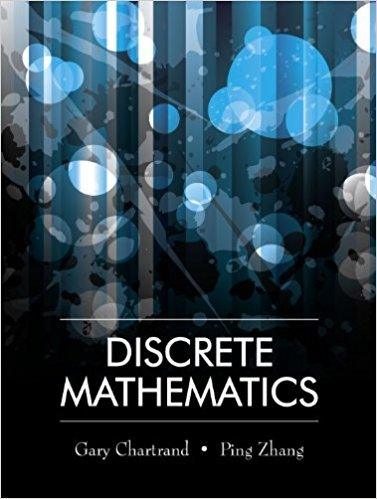×
×

# Solutions for Chapter 5.2: Equivalence Relations## Full solutions for Discrete Mathematics | 1st Edition

ISBN: 9781577667308Solutions for Chapter 5.2: Equivalence Relations

Solutions for Chapter 5.2
4 5 0 296 Reviews
12
2
##### ISBN: 9781577667308

This textbook survival guide was created for the textbook: Discrete Mathematics, edition: 1. Chapter 5.2: Equivalence Relations includes 17 full step-by-step solutions. Since 17 problems in chapter 5.2: Equivalence Relations have been answered, more than 12945 students have viewed full step-by-step solutions from this chapter. Discrete Mathematics was written by and is associated to the ISBN: 9781577667308. This expansive textbook survival guide covers the following chapters and their solutions.

Key Math Terms and definitions covered in this textbook
• Big formula for n by n determinants.

Det(A) is a sum of n! terms. For each term: Multiply one entry from each row and column of A: rows in order 1, ... , nand column order given by a permutation P. Each of the n! P 's has a + or - sign.

• Cholesky factorization

A = CTC = (L.J]))(L.J]))T for positive definite A.

• Determinant IAI = det(A).

Defined by det I = 1, sign reversal for row exchange, and linearity in each row. Then IAI = 0 when A is singular. Also IABI = IAIIBI and

• Factorization

A = L U. If elimination takes A to U without row exchanges, then the lower triangular L with multipliers eij (and eii = 1) brings U back to A.

• Identity matrix I (or In).

Diagonal entries = 1, off-diagonal entries = 0.

• Independent vectors VI, .. " vk.

No combination cl VI + ... + qVk = zero vector unless all ci = O. If the v's are the columns of A, the only solution to Ax = 0 is x = o.

• Iterative method.

A sequence of steps intended to approach the desired solution.

• Kronecker product (tensor product) A ® B.

Blocks aij B, eigenvalues Ap(A)Aq(B).

• Krylov subspace Kj(A, b).

The subspace spanned by b, Ab, ... , Aj-Ib. Numerical methods approximate A -I b by x j with residual b - Ax j in this subspace. A good basis for K j requires only multiplication by A at each step.

• Length II x II.

Square root of x T x (Pythagoras in n dimensions).

• Linear combination cv + d w or L C jV j.

• Markov matrix M.

All mij > 0 and each column sum is 1. Largest eigenvalue A = 1. If mij > 0, the columns of Mk approach the steady state eigenvector M s = s > O.

• Nullspace matrix N.

The columns of N are the n - r special solutions to As = O.

• Nullspace N (A)

= All solutions to Ax = O. Dimension n - r = (# columns) - rank.

• Permutation matrix P.

There are n! orders of 1, ... , n. The n! P 's have the rows of I in those orders. P A puts the rows of A in the same order. P is even or odd (det P = 1 or -1) based on the number of row exchanges to reach I.

• Projection p = a(aTblaTa) onto the line through a.

P = aaT laTa has rank l.

• Singular matrix A.

A square matrix that has no inverse: det(A) = o.

• Subspace S of V.

Any vector space inside V, including V and Z = {zero vector only}.

• Symmetric factorizations A = LDLT and A = QAQT.

Signs in A = signs in D.

• Wavelets Wjk(t).

Stretch and shift the time axis to create Wjk(t) = woo(2j t - k).

×